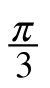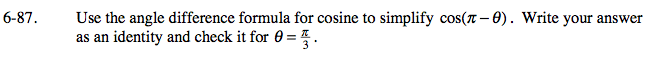### Home > PC > Chapter 6 > Lesson 6.2.2 > Problem6-87

6-87.

Use the angle difference formula for cosine to simplify cos(πθ). Write your answer as an identity and check it for θ =. Homework Help ✎cos(πθ) = cosπ cosθ + sinπ sinθ
= −1cosθ + 0 · sinθ
cos(πθ) = −cosθ